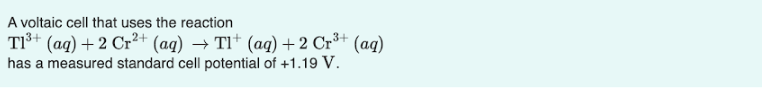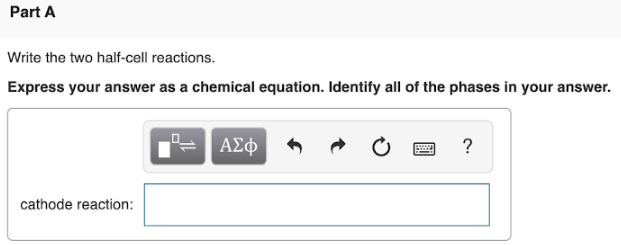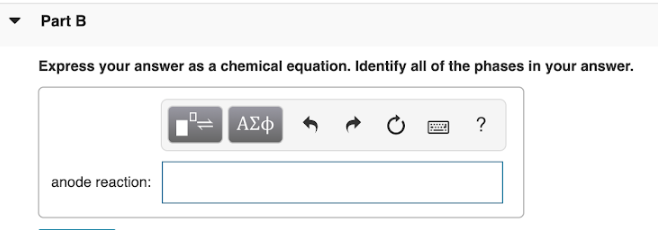# A voltaic cell that uses the reaction Tl^3+(aq) + 2Cr^2+(aq) → Tl^+ (aq) + 2 Cr^3+(aq) has measured standard cell potential of + 1.19 V. Write the two half-cell reactions. Express your answer as a chemical equation. Identify all the phases in your answer.